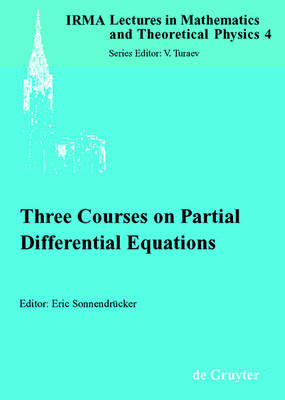•# Three Courses on Partial Differential Equations - IRMA Lectures in Mathematics & Theoretical Physics (Paperback)

(editor)
£63.50
Paperback 168 Pages / Published: 10/11/2003
• We can order this

Usually dispatched within 3 weeks

Modeling, in particular with partial differential equations, plays an ever growing role in the applied sciences. Hence its mathematical understanding is an important issue for today's research. This book provides an introduction to three different topics in partial differential equations arising from applications. The subject of the first course by Michel Chipot (Zurich) is equilibrium positions of several disks rolling on a wire. In particular, existence and uniqueness of and the exact position for an equilibrium are discussed. The second course by Josselin Garnier (Toulouse) deals with problems arising from acoustics and geophysics where waves propagate in complicated media, the properties of which can only be described statistically. It turns out that if the different scales presented in the problem can be separated, there exists a deterministic result. The third course by Otared Kavian (Versailles St.-Quentin) is devoted to so-called inverse problems where one or several parameters of a partial differential equation need to be determined by using, for instance, measurements on the boundary of the domain. The question that arises naturally is what information is necessary to determine the unknown parameters. This question is answered in different settings. The text is addressed to students and researchers with a basic background in partial differential equations.

Publisher: De Gruyter
ISBN: 9783110179583
Number of pages: 168
Weight: 335 g
Dimensions: 240 x 170 x 9 mm